Do you like the article?
Share it with others —
Use new possibilities of MetaTrader 5

#### Similar articles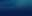# Learn how to design a trading system by Gator Oscillator4 8020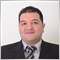### Introduction

We will cover this indicator as much as we can through the following topics:

I need to mention here that you have to test any mentioned strategy before using it to make sure that it will be useful and profitable for you as there is nothing suitable for all people and the main objective here is educational only to learn the main concept and the root behind the indicator and also there is a piece of advice I need to mention here and it is that you need to try writing codes of this article and others by yourself if you want to improve your programming skills.

Disclaimer: All information provided 'as is' only for educational purposes and is not prepared for trading purposes or advice. The information does not guarantee any kind of result. If you choose to use these materials on any of your trading accounts, you will do that at your own risk and you will be the only responsible.

### Gator Oscillator definition

In this part, we will identify the Gator Oscillator indicator in more detail to understand and recognize the main concept behind it and use it in a proper and effective way. The Gator Oscillator indicator was created by Bill Williams to help us identify if the market is trending or ranging if there is a trend how much this trend can continue in terms of momentum, in addition to giving the timing of entering or exiting a trade. As the same as we all know that these two things are very important in trading, The Gator Oscillator is based on the Alligator indicator and you can read my previous article Learn how to design a trading system by Alligator for more details about this Alligator indicator.

The calculation of the Gator indicator is the same as we will see showing how much convergence and divergence of balance lines of the Alligator indicator. The following is for the calculation of the Gator indicator:

• Step one: we need to calculate the median price:

Median Price = (High + Low) /2

• Step two: we need to calculate the Alligator Jaws, the Alligator Teeth, and Alligator Lips:
Alligator Jaw = SMMA (Median Price, 13,8)
Alligator Teeth = SMMA (Median Price, 8, 5)
Alligator Lips = SMMA (Median Price, 5, 3)
• Where:

Median Price: the type of price.

High: the highest price value.

Low: the lowest price value.

SMMA: the smoothed moving average is a type of moving average, it is a smoothing way for data, period, and shift if it exists. If we said that SMMA (Median Price, 13, 5), means that the SMMA which is the smoothed moving average derived from the median price as a type of data, the smoothing period is 13, and the shift to future will be 5.

Alligator Jaws: the blue line of the Alligator indicator.

Alligator Teeth: the red line of the Alligator indicator.

Alligator Lips: the green line of the alligator indicator.

The previous calculation produces the Gator Oscillator indicator but we do not need to calculate it manually as it is built into the MetaTrader 5 and all we need is to choose it from other available technical indicators and insert it into the chart the same as the following.

While opening the MetaTrader 5, choose the Insert tab --> Indicators --> Bill Williams --> Gator Oscillator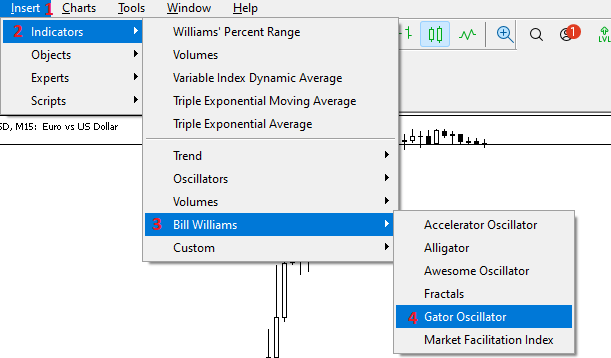After selecting Gator Oscillator we will find the window of Gator parameters the same as the following: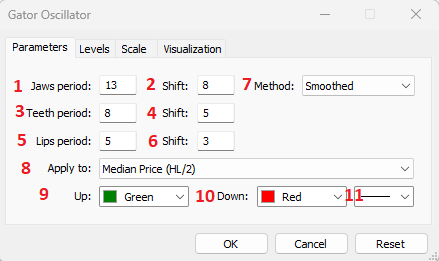In the previous figure, we have parameters of the Gator Oscillator indicator to determine desired settings of the indicator and it is the same as the following:

1. To determine the jaws' period.
2. To determine horizontal shift for Jaws.
3. To determine the teeth period.
4. To determine horizontal shift for teeth.
5. To determine lips' period.
6. To determine horizontal shift for lips.
7. To determine the preferred method of the average.
8. To determine the type of price that will be used in the calculation of the Gator.
9. To determine the color of Gator up values.
10. To determine the color of Gator down values.
11. To determine the thickness of the bars of the Gator.

After determining all preferred parameters of the Gator Oscillator indicator we will find the indicator is attached to the chart in the lower part of the chart the same as the following: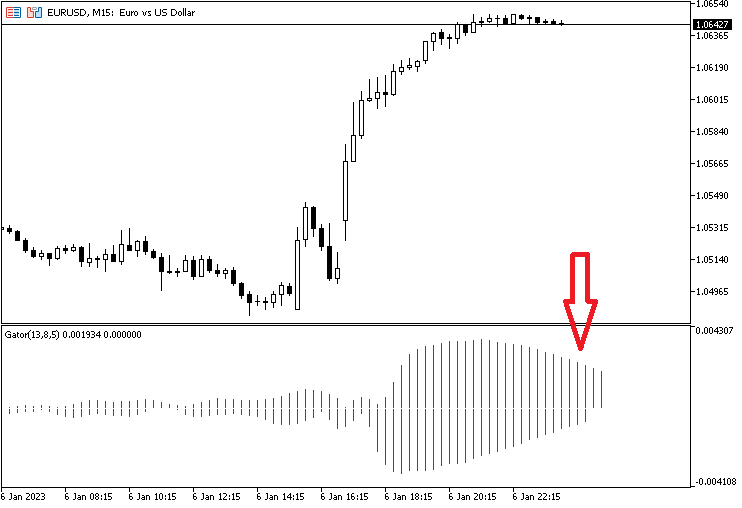As we can in the previous chart we have the indicator with up and down values of the Gator Oscillator which can be seen clearly that we have up values above zero and down values below zero. We have also green and red bars based on the relation between each bar and its previous one the current bar is greater than the previous we will see that the current one is green and vice versa if the current is lower than the previous we will find the current is red.

### Gator Oscillator strategy

In this topic, I will share with you some simple strategies that can be used by the Gator Oscillator indicator for learning purposes only. You must test any mentioned strategy before using it for a real account to make sure that it will be useful for you.

Strategy one: Gator Status Strategy:

Based on this strategy, we need to identify the Gator status based on the bars of the indicator. According to that, we will have four Gator Statuses. If we have both bars red, the status of the Gator will be a sleeping phase. If we have both bars green, it will be an eating phase. If we have both bars green after red, it will be the awakening phase. If we have both bars red after green, it will be a sated phase.

So, Simply,

Both bars red ==> Sleeping phase

Both bars ==> Eating phase

Both bars green after red ==> Awakening phase

Both bars red after green ==> Sated phase

Strategy two: Gator signals:

According to this strategy we need to get signals based on the Gator indicator. If the gator is in the awakening phase, we need to get a signal of finding a good entry. If the Gator indicator is in the eating phase, we need to get a signal of holding the current position. If the Gator indicator is in the sated phase, we need to get find a good exit. If the Gator status is something else, we need to get nothing.

Simply,

The Gator indicator = Awakening phase ==> Find a good entry.

The Gator Indicator = Eating phase ==> Hold current position.

The Gator indicator = Sated phase ==> Find a good exit.

If the Gator indicator status = something else ==> Do nothing.

Strategy three: Gator with MA:

According to this strategy, we will combine the Gator signal with the moving average signal. If we have double green bars and the closing price is above the moving average value, it will be a find good buy position signal. the other scenario, if the Gator indicator has double red bars and the closing price is below the moving average value, it will be a find good sell position. Or, if we have anything else, we need to do nothing.

Simply,

Double green bars and the closing price > the moving average ==> Find a good buy position.

Double red bars and the closing price < the moving average value ==> Find a good sell position.

Anything else ==> Do nothing

### Gator Oscillator blueprint

In this part, we will create step-by-step blueprints for every mentioned strategy to help us to create our trading system effectively and easily. I believe that this step is very important and essential for trading system development as it will save much time even if it takes time to create as it will let you avoid forgetting any important step and repeating tasks to do things well. We will work on understanding what we need to let the computer do for us by organizing our ideas in clear steps.

Strategy one: Gator Status Identifier:

Based on the concept behind this strategy, we need the computer or to create an expert advisor that can be used to check some values of the Gator indicator every tick automatically which are the current up, the previous two up of the current one, and the current down, and the previous two down of the current one. After this checking, we need the expert to determine the position of each value and perform the following comparison, the first one is about comparing the values of the current and previous Gator up and determining which one is greater than the other. the second one is about comparing values of the current and previous Gator down and determining which one is greater than the other. The result of this comparison will be our desired signals for identifying the Gator status.

If the current up value is smaller than the previous one and the current down value is greater than the previous one, we need the expert or the trading system to return a signal of the sleeping phase as a comment on the chart. In another case, if the current up value is greater than the previous one and at the same time the current down value is smaller than the previous one, we need the trading system to return a signal of the eating phase as a comment on the current. In the third case, if the first previous up value is smaller than the second previous one and the first previous down value is greater than the second previous down one and at the same time, the current up value is greater than the first previous one and the current down value is smaller than the first previous one, we need the trading system to return a comment on the chart with the awakening phase signal. In the fourth and last status, if the first previous up value is greater than the second one and the first previous down value is smaller than the second previous one and at the same time, the current op value is smaller than the first previous one and the current down value is greater than the first previous one, we need the trading system to return a comment on the chart with a sated phase signal.

The following is a simple graph for a blueprint of this trading system: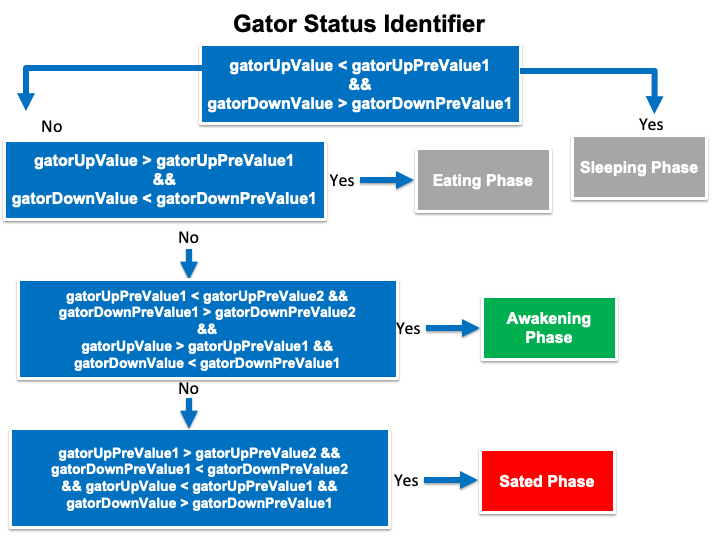Strategy two: Gator signals:

According to the main idea of this trading strategy, we need to create a trading system that can be used to return a signal of good timing of entry, exit, or holding the current position. To do that, we need the trading system to continuously check values of current up and two previous up in addition to, the current down and two previous down also to get the signal based on the Gator status.

The first signal that we need the trading system to return is (Find a good entry) as a comment on the chart after checking the Gator values and finding that there was an awakening phase because the first previous up is smaller than the second previous one and the first previous down value is greater than the second one and at the same time, the current up value is greater than the first previous one and the current down value is smaller than the first previous one.

The second signal that we need to get by the trading system is (Hold current position) as a comment on the chart after checking the Gator values and finding that there was an eating phase because the current up value is greater than the first previous one and the current down value is smaller than the first previous one.

The third signal that we need to get by this trading system is (Find a good exit) as a comment on the chart after checking the Gator values and finding that there was a sated phase because the first previous up value is greater than the second previous one and the first previous down value is greater than the first previous one.

The final thing that we need in the trading system is to do nothing if there is anything except what we mentioned in the previous three signals. The following is the blueprint of this trading system: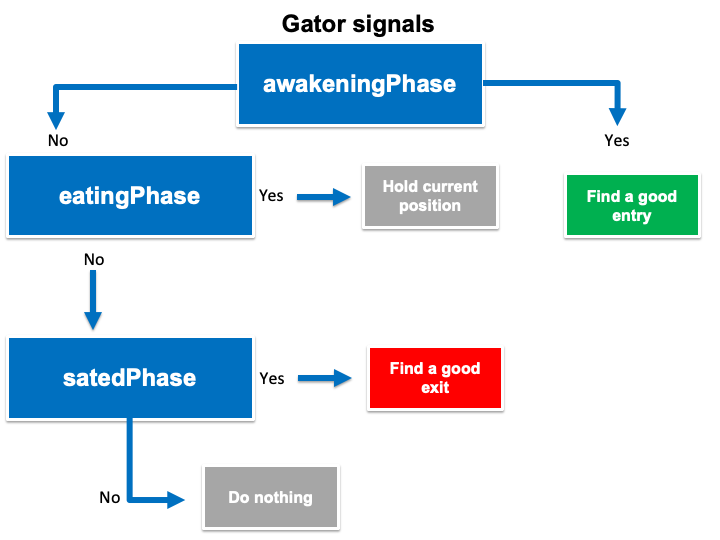Strategy three: Gator with MA:

According to the trading strategy, we need to get a good time to find buy or sell positions based on the Gator indicator, the closing price, and the moving average the same as we learned in the strategy section, the following is for how to let the computer do that.

The first signal that we need the trading system to return is (Find a good buy position) when checking the Gator values and finding that the current up is greater than the first previous one and the first previous up is greater than the second previous one and at the same time, the current down is smaller than the first previous one and the first previous down is smaller than the second previous one, this means that we have now double green bars. Then, the closing price is greater than the moving average value.

The second signal is to get (Find a good sell position) when checking the Gator and finding that the current up is smaller than the first previous one and the first previous up is smaller than the second previous one and at the same time, the current down value is greater than the first previous one and the first previous down is greater than the second previous one, this means that we have double red bars. Then, the closing price is smaller than the moving average.

The third thing we need the trading system to return nothing if there is something else. The following is the blueprint of this trading system: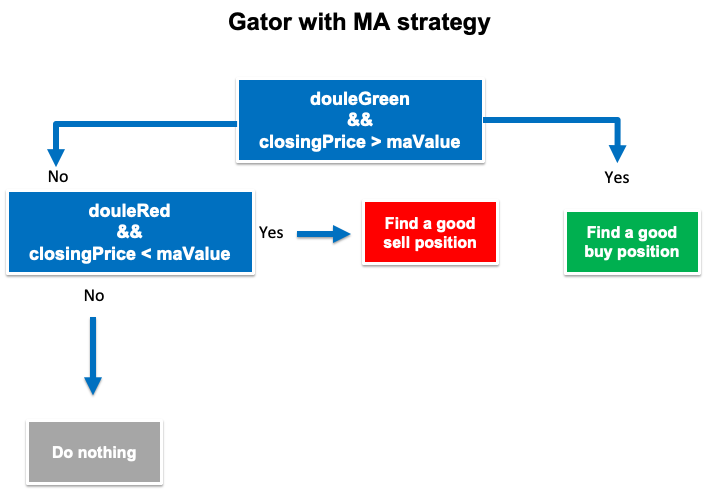Now, we came to the most interesting topic in this article to create our trading system for every mentioned strategy. This trading system can help us to trade effectively we will start to create a simple trading system to be used as a base for our strategies.

The "Simple Gator Oscillator System" is created to return a comment on the chart with the current up value and down value of the Gator indicator. The following steps are for creating this trading system:

Create Arrays of upGator and downGator by using a double function which is one of the real types to return values with fractions.

```   double upGatorArray[];
double downGatorArray[];```

Sorting data in these arrays by using the "ArraySetAsSeries" function. Its parameters:

```   ArraySetAsSeries(upGatorArray,true);
ArraySetAsSeries(downGatorArray,true);```

Creating an integer variable for gatorDef and defining the Gator Oscillator by using the "iGator" function. to return the indicator handle Its parameters:

• symbol: to determine the symbol name, we'll use _SYMBOL to be applied for the current symbol.
• period: to determine the period, we'll use _PERIOD to be applied for the current time frame.
• jaw_period: to determine the desired period of the jaws' calculation, we'll use (13).
• jaw_shift: to determine the jaws' horizontal shift if needed. We'll use (8).
• teeth_period: to determine the period of the teeth calculation. We'll use (8).
• teeth_shift: to determine the teeth' horizontal shift if needed. We'll use (5).
• lips_period: to determine the period of the lips' calculation. We'll use (5).
• lips_shift: to determine the lips' horizontal shift if needed.  We'll use (3).
• ma_method: to determine the type of moving average type. We'll use (MODE_SMMA).
• applied_price: to determine the type of applied price in the calculation. We'll use (PRICE_MEDIAN).
`int gatorDef=iGator(_Symbol,_Period,13,8,8,5,5,3,MODE_SMMA,PRICE_MEDIAN);`

Defining data and storing results by using the "CopyBuffer" function for both upGatorArray and downGatorArray. Its parameters:

• indicator_handle: to determine the indicator handle, we will use (gatorDef).
• buffer_num: to determine the indicator buffer number, we will use (0 for upGator), (2 for downGator).
• start_pos: to determine the start position, we will determine (0).
• count: to determine the amount to copy, we will use (3).
• buffer[]: to determine the target array to copy, we will use (upGatorArray, downGatorArray).
```   CopyBuffer(gatorDef,0,0,3,upGatorArray);
CopyBuffer(gatorDef,2,0,3,downGatorArray);```

Getting values of upGator and downGator after creating double variables for them. Then, we will use the (NormalizeDouble) function for rounding purposes.

• value: We'll use upGatorArray for the current value.
• digits: We'll use (6) for the digits after the decimal point.
```   double gatorUpValue=NormalizeDouble(upGatorArray,6);
double gatorDownValue=NormalizeDouble(downGatorArray,6);```

Using the (Comment) function to appear the values of the current upGator and downGator.

```   Comment("gatorUpValue = ",gatorUpValue,"\n",
"gatorDownValue = ",gatorDownValue);```

The following is the full code of this trading system:

```//+------------------------------------------------------------------+
//|                               Simple Gator Oscillator System.mq5 |
//|                                  Copyright 2022, MetaQuotes Ltd. |
//|                                             https://www.mql5.com |
//+------------------------------------------------------------------+
#property version   "1.00"
//+------------------------------------------------------------------+
void OnTick()
{
double upGatorArray[];
double downGatorArray[];
ArraySetAsSeries(upGatorArray,true);
ArraySetAsSeries(downGatorArray,true);
int gatorDef=iGator(_Symbol,_Period,13,8,8,5,5,3,MODE_SMMA,PRICE_MEDIAN);
CopyBuffer(gatorDef,0,0,3,upGatorArray);
CopyBuffer(gatorDef,2,0,3,downGatorArray);
double gatorUpValue=NormalizeDouble(upGatorArray,6);
double gatorDownValue=NormalizeDouble(downGatorArray,6);
Comment("gatorUpValue = ",gatorUpValue,"\n",
"gatorDownValue = ",gatorDownValue);
}
//+------------------------------------------------------------------+```

After writing the previous lines of code we will compile it, making sure that there are no errors then we will find this expert in the navigator window under the Expert Advisors folder in the MetaTrader 5 trading terminal the same as the following: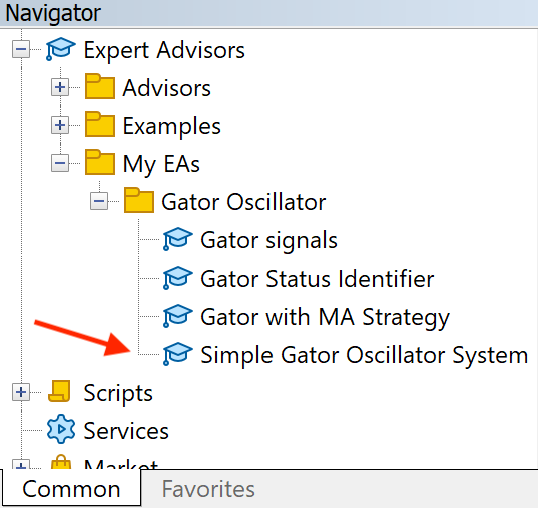By dragging and dropping the expert on the desired chart, we will find the window of this EA the same as the following: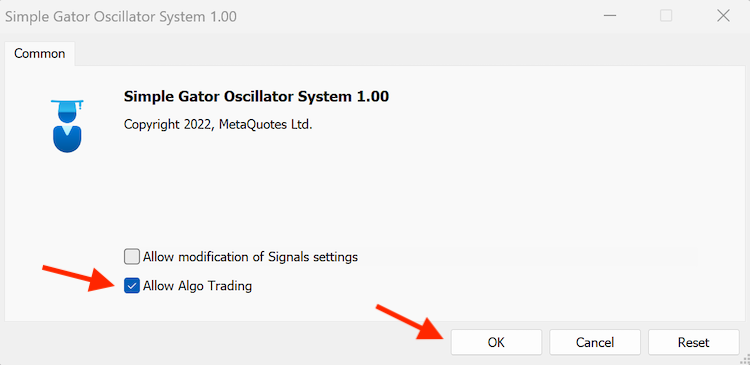After ticking next to (Allow Algo Trading) and pressing (OK), we can find the EA is attached to the chart the same of the following: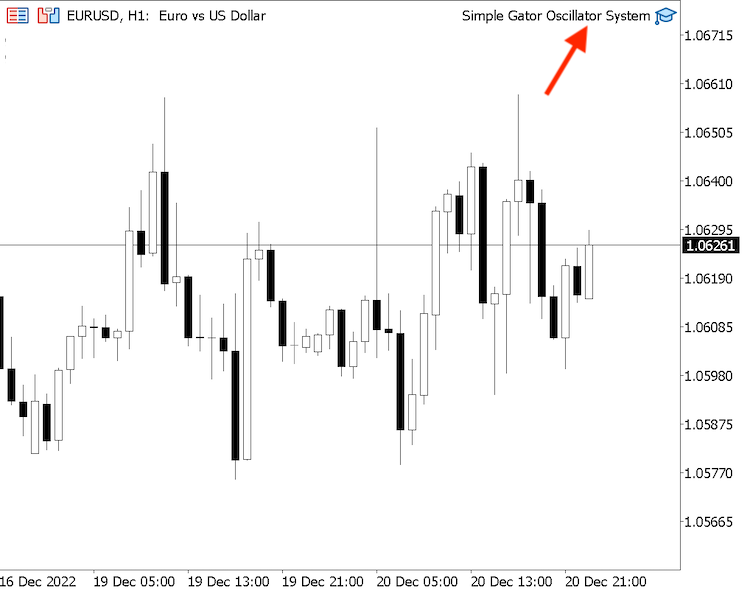Now, we're ready to receive signals of this trading system the same of the following example from testing: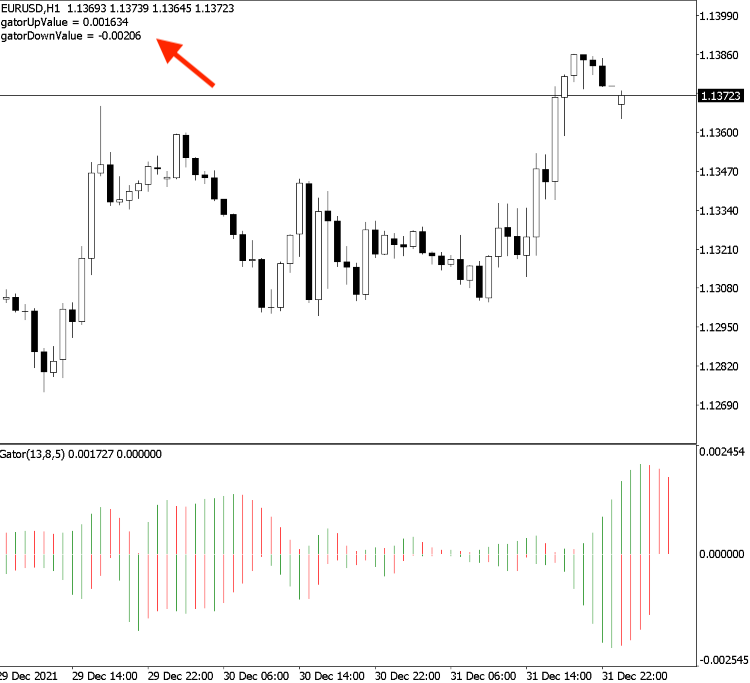Strategy one: Gator Status Identifier:

Based on this strategy the following is for the full block of code to create it:

```//+------------------------------------------------------------------+
//|                                      Gator Status Identifier.mq5 |
//|                                  Copyright 2022, MetaQuotes Ltd. |
//|                                             https://www.mql5.com |
//+------------------------------------------------------------------+
#property version   "1.00"
//+------------------------------------------------------------------+
void OnTick()
{
double upGatorArray[];
double downGatorArray[];
ArraySetAsSeries(upGatorArray,true);
ArraySetAsSeries(downGatorArray,true);
int gatorDef=iGator(_Symbol,_Period,13,8,8,5,5,3,MODE_SMMA,PRICE_MEDIAN);
CopyBuffer(gatorDef,0,0,5,upGatorArray);
CopyBuffer(gatorDef,2,0,5,downGatorArray);
double gatorUpValue=NormalizeDouble(upGatorArray,6);
double gatorUpPreValue1=NormalizeDouble(upGatorArray,6);
double gatorUpPreValue2=NormalizeDouble(upGatorArray,6);
double gatorDownValue=NormalizeDouble(downGatorArray,6);
double gatorDownPreValue1=NormalizeDouble(downGatorArray,6);
double gatorDownPreValue2=NormalizeDouble(downGatorArray,6);
if(gatorUpValue<gatorUpPreValue1&&gatorDownValue>gatorDownPreValue1)
{
Comment("Sleeping Phase");
}
else
if(gatorUpValue>gatorUpPreValue1&&gatorDownValue<gatorDownPreValue1)
{
Comment("Eating Phase");
}
if(gatorUpPreValue1<gatorUpPreValue2&&gatorDownPreValue1>gatorDownPreValue2&&
gatorUpValue>gatorUpPreValue1&&gatorDownValue<gatorDownPreValue1)
{
Comment("Awakening Phase");
}
else
if(
gatorUpPreValue1>gatorUpPreValue2&&gatorDownPreValue1<gatorDownPreValue2&&
gatorUpValue<gatorUpPreValue1&&gatorDownValue>gatorDownPreValue1)
{
Comment("Sated Phase");
}
}
//+------------------------------------------------------------------+
```

Differences in this code:

Defining and getting the three last values of upGator

```   double gatorUpValue=NormalizeDouble(upGatorArray,6);
double gatorUpPreValue1=NormalizeDouble(upGatorArray,6);
double gatorUpPreValue2=NormalizeDouble(upGatorArray,6);```

Defining and getting the three last values of downGator

```   double gatorDownValue=NormalizeDouble(downGatorArray,6);
double gatorDownPreValue1=NormalizeDouble(downGatorArray,6);
double gatorDownPreValue2=NormalizeDouble(downGatorArray,6);```

Conditions of the strategy:

In the case of the sleeping phase,

```   if(gatorUpValue<gatorUpPreValue1&&gatorDownValue>gatorDownPreValue1)
{
Comment("Sleeping Phase");
}```

In the case of the eating phase,

```   else
if(gatorUpValue>gatorUpPreValue1&&gatorDownValue<gatorDownPreValue1)
{
Comment("Eating Phase");
}```

In the case of the awakening phase,

```   if(gatorUpPreValue1<gatorUpPreValue2&&gatorDownPreValue1>gatorDownPreValue2&&
gatorUpValue>gatorUpPreValue1&&gatorDownValue<gatorDownPreValue1)
{
Comment("Awakening Phase");
}```

In the case of the sated phase,

```   else
if(
gatorUpPreValue1>gatorUpPreValue2&&gatorDownPreValue1<gatorDownPreValue2&&
gatorUpValue<gatorUpPreValue1&&gatorDownValue>gatorDownPreValue1)
{
Comment("Sated Phase");
}```

After compiling this code without any errors and executing the EA, we'll find it is attached to the chart the same as the following: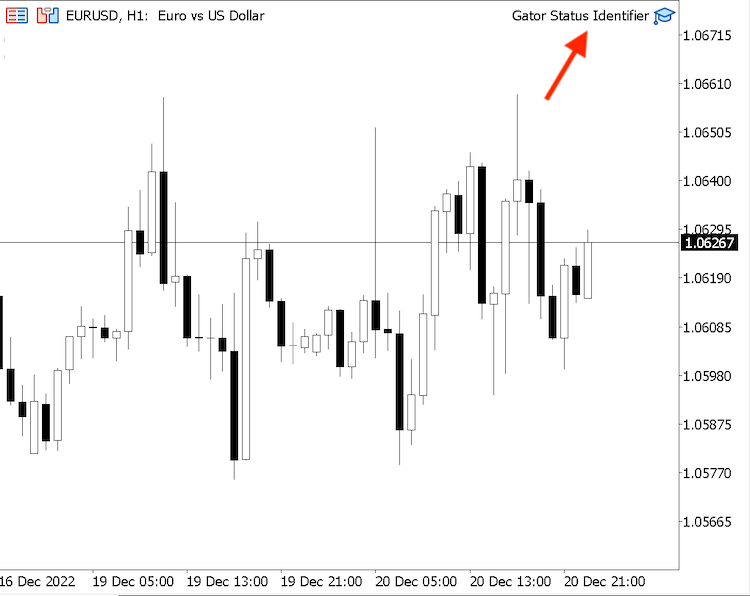As we can see in the top right corner of the previous chart that we have the Gator Status Identifier EA is attached to the chart.

We can find signals based on this strategy the same as the following from testing:

In the case of a sleeping signal: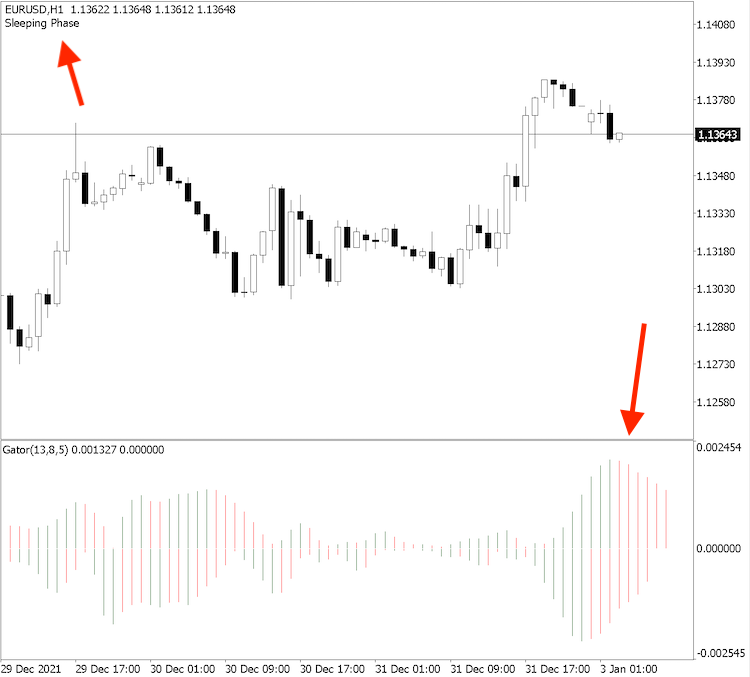As we can see on the previous chart in the top left corner we have a signal of the sleeping phase based on this strategy.

In the case of the eating phase: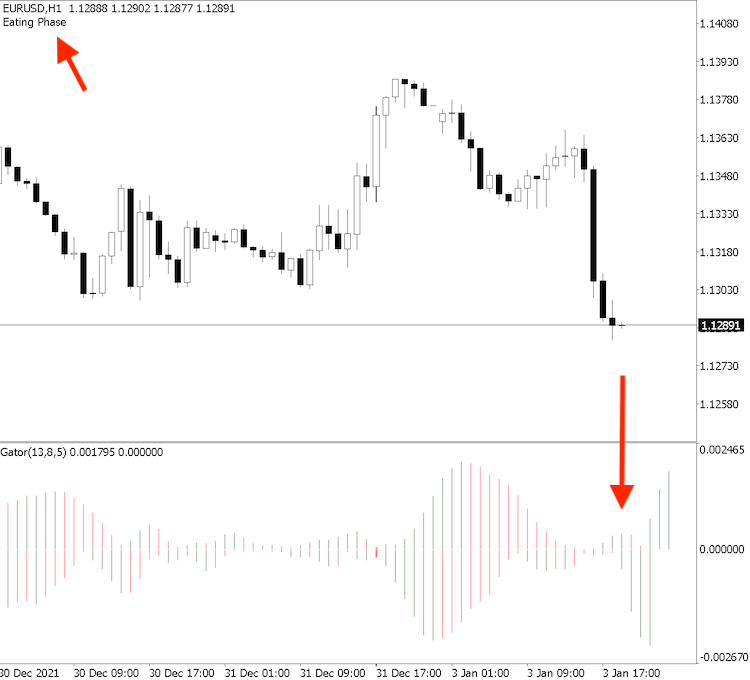Based on the previous chart we can find in the top left corner that we have an eating phase signal depending on his strategy.

In the case of the awakening phase: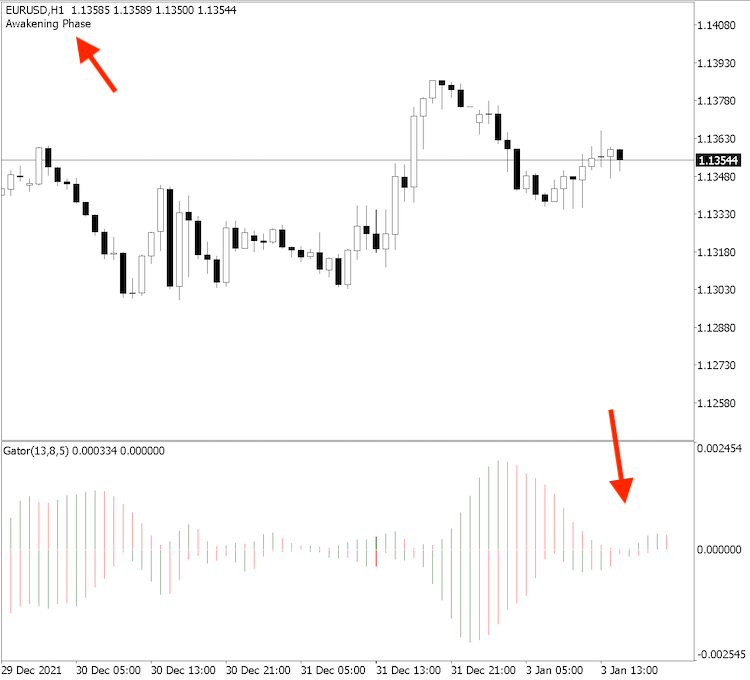As we can see through the previous chart we have an awakening phase signal based on the Gator Status Identifier strategy.

In the case of the sated phase: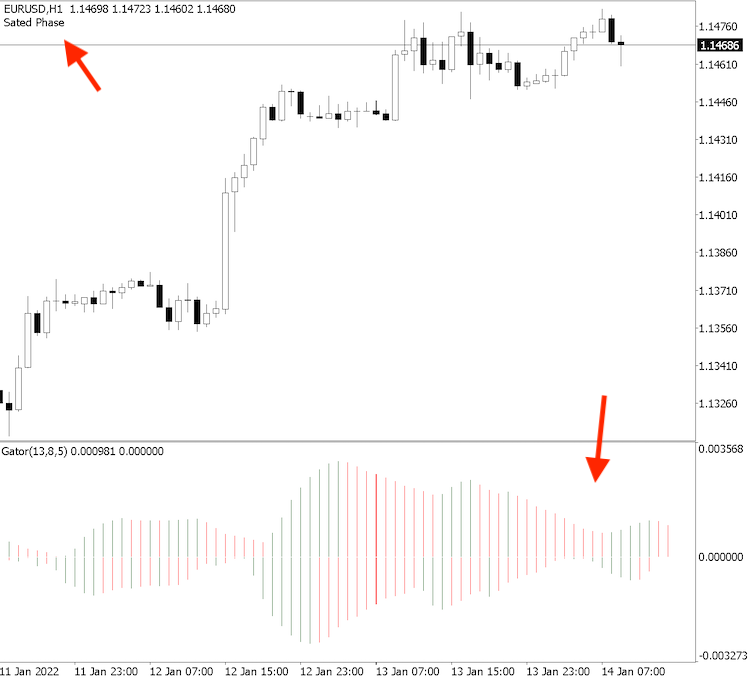As we can see in the previous figure we have a sated phase in the top left corner.

Strategy two: Gator signals strategy:

The following is for the full code to create a trading system based on this strategy.

```//+------------------------------------------------------------------+
//|                                                Gator signals.mq5 |
//|                                  Copyright 2022, MetaQuotes Ltd. |
//|                                             https://www.mql5.com |
//+------------------------------------------------------------------+
#property version   "1.00"
//+------------------------------------------------------------------+
void OnTick()
{
double upGatorArray[];
double downGatorArray[];
ArraySetAsSeries(upGatorArray,true);
ArraySetAsSeries(downGatorArray,true);
int gatorDef=iGator(_Symbol,_Period,13,8,8,5,5,3,MODE_SMMA,PRICE_MEDIAN);
CopyBuffer(gatorDef,0,0,3,upGatorArray);
CopyBuffer(gatorDef,2,0,3,downGatorArray);
double gatorUpValue=NormalizeDouble(upGatorArray,6);
double gatorUpPreValue1=NormalizeDouble(upGatorArray,6);
double gatorUpPreValue2=NormalizeDouble(upGatorArray,6);
double gatorDownValue=NormalizeDouble(downGatorArray,6);
double gatorDownPreValue1=NormalizeDouble(downGatorArray,6);
double gatorDownPreValue2=NormalizeDouble(downGatorArray,6);
bool awakeningPhase = gatorUpPreValue1<gatorUpPreValue2&&gatorDownPreValue1>gatorDownPreValue2&&
gatorUpValue>gatorUpPreValue1&&gatorDownValue<gatorDownPreValue1;
bool eatingPhase = gatorUpValue>gatorUpPreValue1&&gatorDownValue<gatorDownPreValue1;
bool satedPhase = gatorUpPreValue1>gatorUpPreValue2&&gatorDownPreValue1<gatorDownPreValue2&&
gatorUpValue<gatorUpPreValue1&&gatorDownValue>gatorDownPreValue1;
if(awakeningPhase)
{
Comment("Find a good entry");
}
else
if(eatingPhase)
{
Comment("Hold current position");
}
else
if(satedPhase)
{
Comment("Find a good exit");
}
else
Comment("");
}
//+------------------------------------------------------------------+```

Differences in this strategy:

Creating a bool variable for the following three phases (awakeningPhase, eatingPhase, and satedPhase);

```   bool awakeningPhase = gatorUpPreValue1<gatorUpPreValue2&&gatorDownPreValue1>gatorDownPreValue2&&
gatorUpValue>gatorUpPreValue1&&gatorDownValue<gatorDownPreValue1;
bool eatingPhase = gatorUpValue>gatorUpPreValue1&&gatorDownValue<gatorDownPreValue1;
bool satedPhase = gatorUpPreValue1>gatorUpPreValue2&&gatorDownPreValue1<gatorDownPreValue2&&
gatorUpValue<gatorUpPreValue1&&gatorDownValue>gatorDownPreValue1;```

Conditions of the strategy:

In the case of the awakening Phase

```   if(awakeningPhase)
{
Comment("Find a good entry");
}```

In the case of the eating Phase

```   else
if(eatingPhase)
{
Comment("Hold current position");
}```

In the case of the sated phase

```      else
if(satedPhase)
{
Comment("Find a good exit");
}```

Others

```         else
Comment("");```

After compiling this code and executing it, we'll find the EA is attached to the chart the same as the following: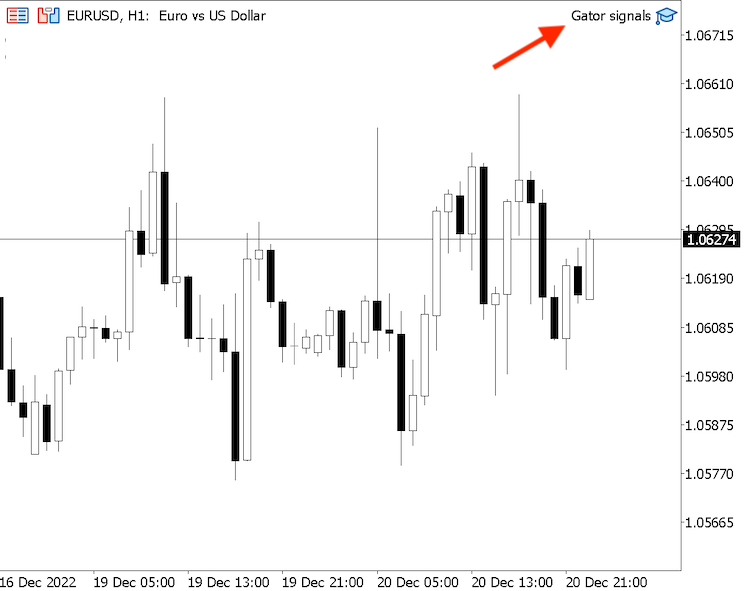As we can see in the top right corner the EA of Gator signals is attached to the chart.

Now, we're ready to receive signals of this strategy and the following are examples from testing:

In the case of the awakening phase;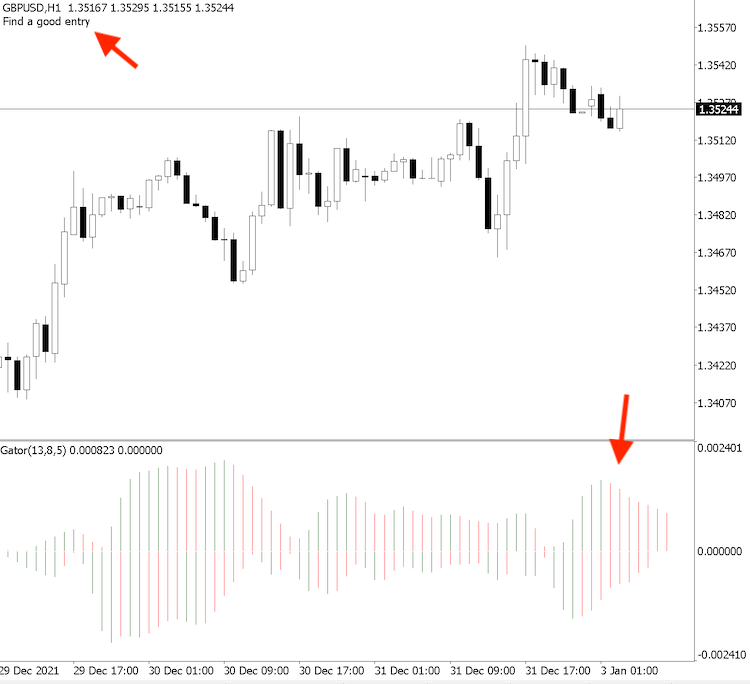As we can see in the previous chart we have a "Find a good entry" signal in the top left corner.

In the case of the eating phase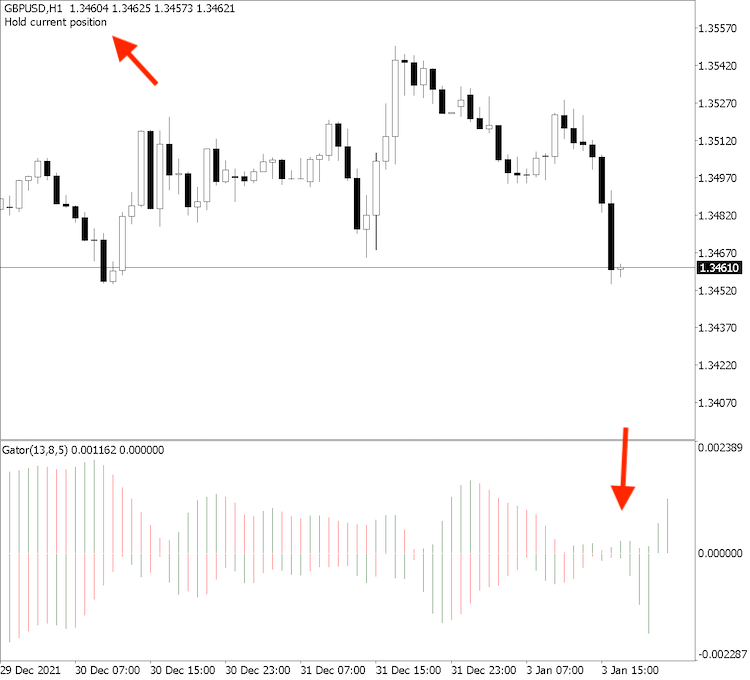As we can see in the previous figure we have a "Hold current position" signal in the top left corner.

In the case of the sated phase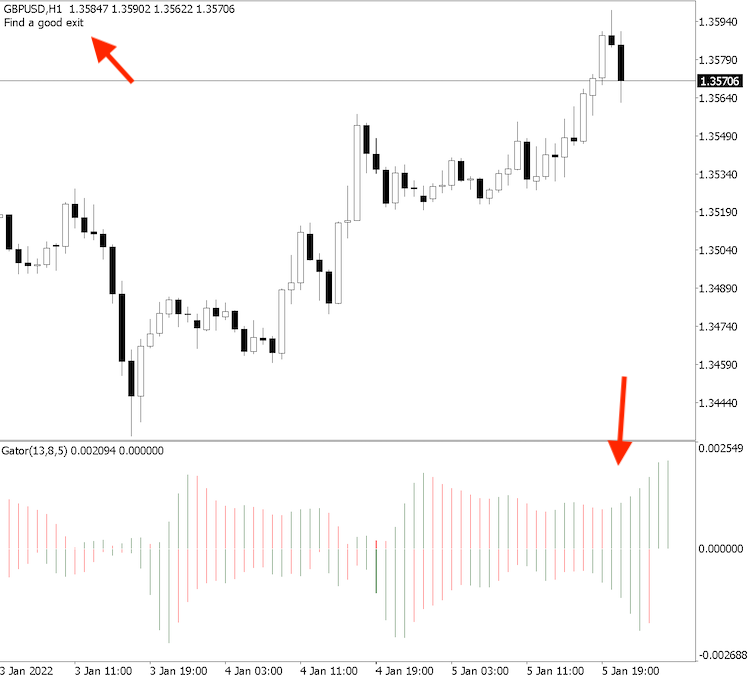As we can see in the previous chart from testing as an example we have a "Find a good exit" signal in the top left corner.

Strategy three: Gator with MA strategy:

The following is the full code for creating a trading system based on this strategy.

```//+------------------------------------------------------------------+
//|                                       Gator with MA strategy.mq5 |
//|                                  Copyright 2022, MetaQuotes Ltd. |
//|                                             https://www.mql5.com |
//+------------------------------------------------------------------+
#property version   "1.00"
//+------------------------------------------------------------------+
void OnTick()
{
double upGatorArray[];
double downGatorArray[];
MqlRates pArray[];
double maArray[];
ArraySetAsSeries(upGatorArray,true);
ArraySetAsSeries(downGatorArray,true);
ArraySetAsSeries(maArray,true);
int gatorDef=iGator(_Symbol,_Period,13,8,8,5,5,3,MODE_SMMA,PRICE_MEDIAN);
int data=CopyRates(_Symbol,_Period,0,13,pArray);
CopyBuffer(gatorDef,0,0,3,upGatorArray);
CopyBuffer(gatorDef,2,0,3,downGatorArray);
double gatorUpValue=NormalizeDouble(upGatorArray,6);
double gatorUpPreValue1=NormalizeDouble(upGatorArray,6);
double gatorUpPreValue2=NormalizeDouble(upGatorArray,6);
double gatorDownValue=NormalizeDouble(downGatorArray,6);
double gatorDownPreValue1=NormalizeDouble(downGatorArray,6);
double gatorDownPreValue2=NormalizeDouble(downGatorArray,6);
double maValue=NormalizeDouble(maArray,5);
double closingPrice=pArray.close;
bool douleGreen = gatorUpValue>gatorUpPreValue1&&gatorUpPreValue1>gatorUpPreValue2&&
gatorDownValue<gatorDownPreValue1&&gatorDownPreValue1<gatorDownPreValue2;
bool douleRed = gatorUpValue<gatorUpPreValue1&&gatorUpPreValue1<gatorUpPreValue2&&
gatorDownValue>gatorDownPreValue1&&gatorDownPreValue1>gatorDownPreValue2;
if(douleGreen&&closingPrice>maValue)
{
}
else
if(douleRed&&closingPrice<maValue)
{
Comment("Find a good sell position");
}
else
Comment("");
}
//+------------------------------------------------------------------+```

Differences in this strategy:

Creating two more arrays for pArray and maArray; we will use the "MqlRates" function for pArray to store information of prices. and the "double" function for maArray;

```   MqlRates pArray[];
double maArray[];```

Sorting data in maArray by using the "ArraySetAsSeries" function;

`ArraySetAsSeries(maArray,true);`

Creating an integer variable for maDef and defining the Moving Average by using the "iMA" function to return the indicator handle and its parameters:

• symbol:  to determine the symbol name. We'll determine (_SYMBOL) to be applied for the current chart.
• period: to determine the period, we'll use _PERIOD to be applied for the current time frame and you can also set (PERIOD_CURRENT) for the same.
• ma_period: to determine the average period, we'll use (13).
• ma_shift: to determine the horizontal shift if needed. We'll set (0) as we do need not to shift the MA.
• ma_method: to determine the moving average type, we'll set EMA (Exponential Moving Average).
• applied_price: to determine the type of used price in the calculation, we'll use the closing price.
`int maDef=iMA(_Symbol,_Period,13,0,MODE_EMA,PRICE_CLOSE);`

Getting historical data of MqlRates by using the "CopyRates" function:

• symbol_name: to determine the symbol name, we'll use (_Symbol) to be applied for the current symbol.
• timeframe: to determine the timeframe ad we will use the (_Period) to be applied for the current time frame.
• start_pos: to determine the starting point or position, we'll use (0) to start from the current position.
• count: to determine the count to copy, we'll use (13).
• rates_array[]: to determine the target of the array to copy, we'll use (pArray).
`int data=CopyRates(_Symbol,_Period,0,13,pArray);`

Defining data and storing results by using the "CopyBuffer" function for the maArray.

`CopyBuffer(maDef,0,0,3,maArray);`

Getting the value of the current exponential moving average and normalizing it.

`double maValue=NormalizeDouble(maArray,5);`

Getting the current value of the closing price.

`double closingPrice=pArray.close;`

Creating bool variables of double green bars and double red bars of the Gator Oscillator indicator.

```   bool douleGreen = gatorUpValue>gatorUpPreValue1&&gatorUpPreValue1>gatorUpPreValue2&&
gatorDownValue<gatorDownPreValue1&&gatorDownPreValue1<gatorDownPreValue2;
bool douleRed = gatorUpValue<gatorUpPreValue1&&gatorUpPreValue1<gatorUpPreValue2&&
gatorDownValue>gatorDownPreValue1&&gatorDownPreValue1>gatorDownPreValue2; ```

Conditions of the strategy:

```   if(douleGreen&&closingPrice>maValue)
{
}```

In the case of selling;

```   else
if(douleRed&&closingPrice<maValue)
{
Comment("Find a good sell position");
}```

Others;

```      else
Comment("");```

After compiling and executing this code to be attached to the desired chart we'll find the EA is attached to the chart the same as the following: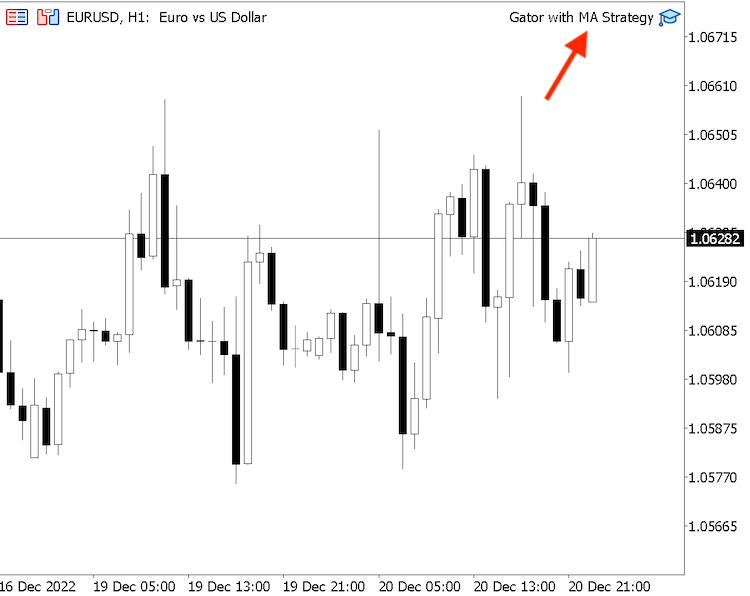As we can see on the top right corner of the chart we have the EA of the Gator with MA attached to the chart.

Now, we're ready to receive signals of this strategy and the following are examples from testing;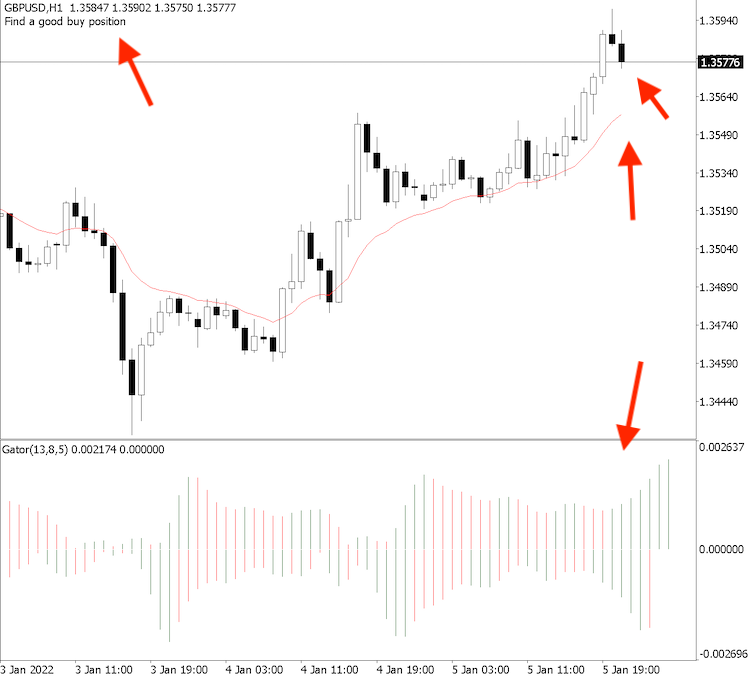As we can see in the previous figure in the top left corner we have a (Find a good buy position) signal.

In the case of selling;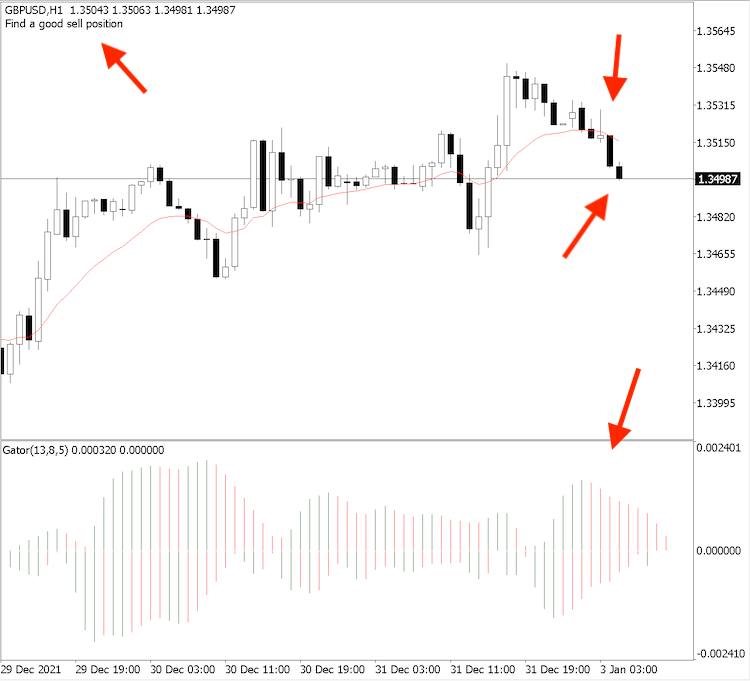As we can see that we have a (Find a good sell position) signal.

Now we learned how we can create trading systems based on different strategies and this approach has to open your eyes to different ideas that you can be applied this is the main objective of this article and series.

### Conclusion

Now, we covered all topics of this article to learn how to design a trading system by Gator Oscillator as we learned what is the Gator Oscillator indicator, how to calculate it, how to use it through three simple trading strategies and they are:

• Gator Status Identifier: this strategy determines what is the status of the Gator Oscillator (awakening, sleeping, eating, sated) based on different conditions.
• Gator signals: to get signals of the timing of suitable decision (Find a good entry, hold current position, or find a good exit) based on different conditions of the Gator Oscillator.
• Gator with MA strategy: to get signals of the timing of buy or sell positions based on the Gator Oscillator with the moving average indicator.

After we learned how to create a step-by-step blueprint to help us to create a trading system for every mentioned strategy effectively and easily. Then, we created a trading system for these strategies to be executed in the MetaTrader5 trading platform by creating their source code in the MQL5 IDE.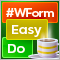DoEasy. Controls (Part 26): Finalizing the ToolTip WinForms object and moving on to ProgressBar development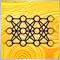Neural networks made easy (Part 32): Distributed Q-Learning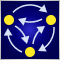MQL5 Wizard techniques you should know (Part 05): Markov Chains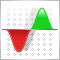Mountain or Iceberg charts